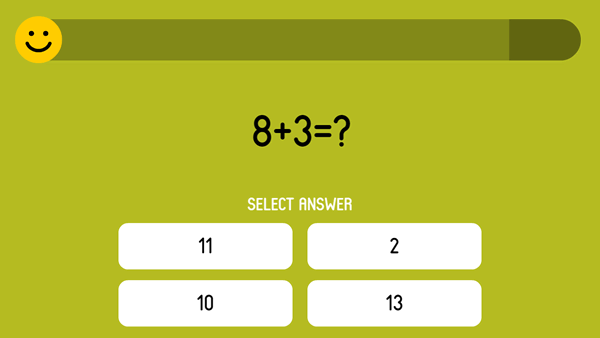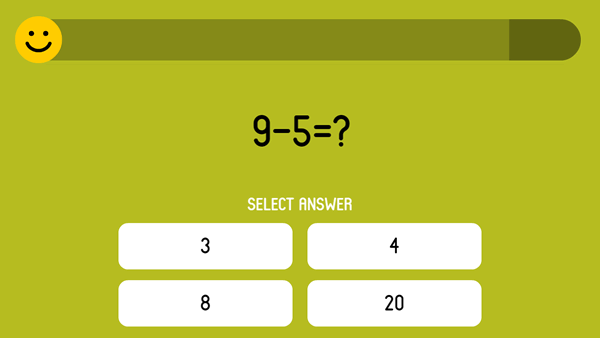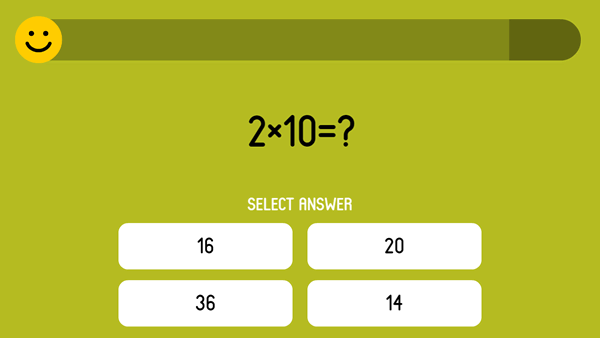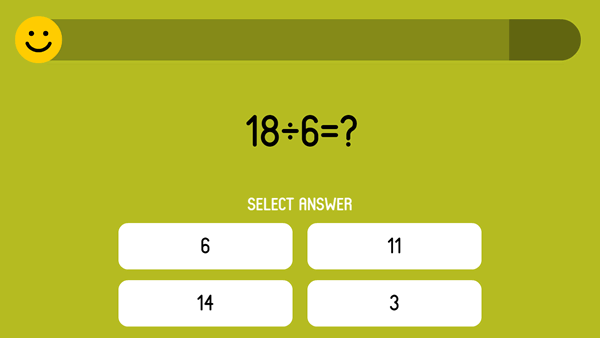# Basic Math Operations Tests

In this group, we gathered the tests basic math operations: plus, minus, multiplication, division. The tests are divided into easy ones – for beginners, intermediate ones – for experienced young math lovers and difficult ones – for advanced ones who can do difficult tasks.

## Test on the arithmetic operation plus

In this test you need to solve tests on the arithmetic operation plus and choose the right answer. You'll get 10 questions online. You'll get a score at the end. Hurry up - the time is limited for each question...## Test on the arithmetic operation minus

In this test you need to solve tests on the arithmetic operation minus and choose the right answer. You'll get 10 questions online. You'll get a score at the end. Hurry up - the time is limited for each questio...## Test on the arithmetic operation multiplication

In this test you need to solve tests on the arithmetic operation multiplication and choose the right answer. You'll get 10 questions online. You'll get a score at the end. Hurry up - the time is limited for eac...## Test on the arithmetic operation division

In this test you need to solve tests on the arithmetic operation division and choose the right answer. You'll get 10 questions online. You'll get a score at the end. Hurry up - the time is limited for each ques...